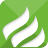# ES6 箭头函数讲解 你正确使用了吗？

```var f = v = > v;
//等同于
var f = function(v){
return v;
}
var sum = (num1,num2) => num1+num2 ;
//等同于
var sum = function(num1,num2){
return num1+num2
}
[1,2,3].map(function (x) {
return x * x;
});
// 箭头函数写法
[1,2,3].map(x => x * x);//简洁了许多```

1、如果箭头函数不需要参数或需要多个参数，就使用一个圆括号代表参数部分。

```const hello = () => 'hello';
const sum = (num1, num2) => num1 + num2;```

2、如果箭头函数的代码块部分多于一条语句，就要使用大括号将它们括起来，并且使用return语句返回。

```const sum = (x, y) => {
let z= 0;
if(x && y){
z= x + y;
}
return z;
}```

3、如果箭头函数直接返回一个对象，必须在对象外面加上括号，否则会报错。

`const getInfo = id => ({ id: id, name: "hello" });`

A、箭头函数的this指向

```// ES6
function foo() {
setTimeout(() => {
console.log('id:', this.id);
}, 100);
}
// ES5
function foo() {
var _this = this;

setTimeout(function () {
console.log('id:', _this.id);
}, 100);
}```

```function test() {
this.s1 = 0;
this.s2 = 0;  // 箭头函数
setInterval(() => this.s1++, 1000);  // 普通函数
setInterval(function () {
this.s2++;
}, 1000);
}

s1 // 1
s2 // 0```

```//普通函数
setInterval(function () {
let _this = this;
_this.s2++;
}, 1000);```

B、箭头函数不能作为构造函数，不能使用new.

```//构造函数如下：
function Person(p){
this.name = p.name;
}
//如果用箭头函数作为构造函数，则如下
var Person = (p) => {
this.name = p.name;
}```

C、箭头函数没有arguments，caller，callee。

```function hello() {
setTimeout(() => {
console.log('args:', arguments);
}, 1000);
}

hello(1,2,3,4)
// args: [1, 2, 3, 4]```

D、箭头函数通过call和apply调用，不会改变this指向，只会传入参数

```let obj2 = {
a: 10,
b: function(n) {
let f = (n) => n + this.a;
return f(n);
},
c: function(n) {
let f = (n) => n + this.a;
let m = {
a: 20
};
return f.call(m,n);
}
};
console.log(obj2.b(1));  // 11
console.log(obj2.c(1)); // 11```

E、 箭头函数没有原型属性

```var a = ()=>{
return 1;
}

function b(){
return 2;
}

console.log(a.prototype);  // undefined
console.log(b.prototype);   // {constructor: ƒ}```

F、箭头函数返回对象时，要加一个小括号

```var func = () => ({ foo: 1 }); //正确
var func = () => { foo: 1 };   //错误```

0.101216s